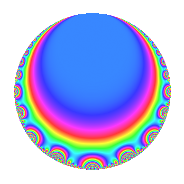# Properties

 Label 308.2.a.bLevel 308 Weight 2 Character orbit 308.a Self dual Yes Analytic conductor 2.459 Analytic rank 0 Dimension 2 CM No Inner twists 1

# Related objects

## Newspace parameters

 Level: $$N$$ = $$308 = 2^{2} \cdot 7 \cdot 11$$ Weight: $$k$$ = $$2$$ Character orbit: $$[\chi]$$ = 308.a (trivial)

## Newform invariants

 Self dual: Yes Analytic conductor: $$2.45939238226$$ Analytic rank: $$0$$ Dimension: $$2$$ Coefficient field: $$\Q(\sqrt{6})$$ Coefficient ring: $$\Z[a_1, a_2, a_3]$$ Coefficient ring index: $$1$$ Fricke sign: $$-1$$ Sato-Tate group: $\mathrm{SU}(2)$

## $q$-expansion

Coefficients of the $$q$$-expansion are expressed in terms of $$\beta = \sqrt{6}$$. We also show the integral $$q$$-expansion of the trace form.

 $$f(q)$$ $$=$$ $$q + \beta q^{3} + 2 q^{5} - q^{7} + 3 q^{9} +O(q^{10})$$ $$q + \beta q^{3} + 2 q^{5} - q^{7} + 3 q^{9} - q^{11} + ( 2 - \beta ) q^{13} + 2 \beta q^{15} + ( 2 - \beta ) q^{17} -2 \beta q^{19} -\beta q^{21} + ( 4 - 2 \beta ) q^{23} - q^{25} + ( -2 + 2 \beta ) q^{29} + ( 4 + \beta ) q^{31} -\beta q^{33} -2 q^{35} + 4 q^{37} + ( -6 + 2 \beta ) q^{39} + ( -2 - 3 \beta ) q^{41} + ( -2 + 2 \beta ) q^{43} + 6 q^{45} + ( -4 - \beta ) q^{47} + q^{49} + ( -6 + 2 \beta ) q^{51} + 4 \beta q^{53} -2 q^{55} -12 q^{57} + 3 \beta q^{59} + ( -2 + 3 \beta ) q^{61} -3 q^{63} + ( 4 - 2 \beta ) q^{65} -6 \beta q^{67} + ( -12 + 4 \beta ) q^{69} + ( -8 - 2 \beta ) q^{71} + ( 10 + \beta ) q^{73} -\beta q^{75} + q^{77} + ( -6 - 2 \beta ) q^{79} -9 q^{81} + ( -12 - 2 \beta ) q^{83} + ( 4 - 2 \beta ) q^{85} + ( 12 - 2 \beta ) q^{87} + 6 q^{89} + ( -2 + \beta ) q^{91} + ( 6 + 4 \beta ) q^{93} -4 \beta q^{95} + ( 10 - 2 \beta ) q^{97} -3 q^{99} +O(q^{100})$$ $$\operatorname{Tr}(f)(q)$$ $$=$$ $$2q + 4q^{5} - 2q^{7} + 6q^{9} + O(q^{10})$$ $$2q + 4q^{5} - 2q^{7} + 6q^{9} - 2q^{11} + 4q^{13} + 4q^{17} + 8q^{23} - 2q^{25} - 4q^{29} + 8q^{31} - 4q^{35} + 8q^{37} - 12q^{39} - 4q^{41} - 4q^{43} + 12q^{45} - 8q^{47} + 2q^{49} - 12q^{51} - 4q^{55} - 24q^{57} - 4q^{61} - 6q^{63} + 8q^{65} - 24q^{69} - 16q^{71} + 20q^{73} + 2q^{77} - 12q^{79} - 18q^{81} - 24q^{83} + 8q^{85} + 24q^{87} + 12q^{89} - 4q^{91} + 12q^{93} + 20q^{97} - 6q^{99} + O(q^{100})$$

## Embeddings

For each embedding $$\iota_m$$ of the coefficient field, the values $$\iota_m(a_n)$$ are shown below.

For more information on an embedded modular form you can click on its label.

Label $$\iota_m(\nu)$$ $$a_{2}$$ $$a_{3}$$ $$a_{4}$$ $$a_{5}$$ $$a_{6}$$ $$a_{7}$$ $$a_{8}$$ $$a_{9}$$ $$a_{10}$$
1.1
 −2.44949 2.44949
0 −2.44949 0 2.00000 0 −1.00000 0 3.00000 0
1.2 0 2.44949 0 2.00000 0 −1.00000 0 3.00000 0
 $$n$$: e.g. 2-40 or 990-1000 Significant digits: Format: Complex embeddings Normalized embeddings Satake parameters Satake angles

## Inner twists

This newform does not admit any (nontrivial) inner twists.

## Atkin-Lehner signs

$$p$$ Sign
$$2$$ $$-1$$
$$7$$ $$1$$
$$11$$ $$1$$

## Hecke kernels

This newform can be constructed as the kernel of the linear operator $$T_{3}^{2} - 6$$ acting on $$S_{2}^{\mathrm{new}}(\Gamma_0(308))$$.